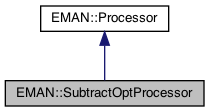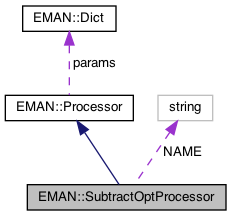EMAN2
EMAN::SubtractOptProcessor Class Reference

Sorry for the pun. More...

`#include <processor.h>`

Inheritance diagram for EMAN::SubtractOptProcessor:[legend]
Collaboration diagram for EMAN::SubtractOptProcessor:[legend]

## Public Member Functions

virtual void process_inplace (EMData *image)
To process an image in-place. More...

virtual EMDataprocess (const EMData *const image)
To proccess an image out-of-place. More...

string get_name () const
Get the processor's name. More...

TypeDict get_param_types () const
Get processor parameter information in a dictionary. More...

string get_desc () const
Get the descrition of this specific processor. More...Public Member Functions inherited from EMAN::Processor
virtual ~Processor ()

virtual void process_list_inplace (vector< EMData * > &images)
To process multiple images using the same algorithm. More...

virtual Dict get_params () const
Get the processor parameters in a key/value dictionary. More...

virtual void set_params (const Dict &new_params)
Set the processor parameters using a key/value dictionary. More...

## Static Public Member Functions

static ProcessorNEW ()Static Public Member Functions inherited from EMAN::Processor
static string get_group_desc ()
Get the description of this group of processors. More...

static void EMFourierFilterInPlace (EMData *fimage, Dict params)
Compute a Fourier-filter processed image in place. More...

static EMDataEMFourierFilter (EMData *fimage, Dict params)
Compute a Fourier-processor processed image without altering the original image. More...

## Static Public Attributes

static const string NAME = "math.sub.optimal"Public Types inherited from EMAN::Processor
enum  fourier_filter_types {
TOP_HAT_LOW_PASS , TOP_HAT_HIGH_PASS , TOP_HAT_BAND_PASS , TOP_HOMOMORPHIC ,
GAUSS_LOW_PASS , GAUSS_HIGH_PASS , GAUSS_BAND_PASS , GAUSS_INVERSE ,
GAUSS_HOMOMORPHIC , BUTTERWORTH_LOW_PASS , BUTTERWORTH_HIGH_PASS , BUTTERWORTH_HOMOMORPHIC ,
KAISER_I0 , KAISER_SINH , KAISER_I0_INVERSE , KAISER_SINH_INVERSE ,
SHIFT , TANH_LOW_PASS , TANH_HIGH_PASS , TANH_HOMOMORPHIC ,
}
Fourier filter Processor type enum. More...Protected Attributes inherited from EMAN::Processor
Dict params

## Detailed Description

Sorry for the pun.

This processor will take a second image and try to filter/scale it to optimally subtract it from the original image. The idea here is that if you have an image with noise plus a linear-filter modified projection, that a good measure of the similarity of the image to the projection would be to try and remove the projection from the image as optimally as possible, then compute the standard deviation of what's left.

Now you might say that if the total energy in the noisy image is normalized then this should be equivalent to just integrating the FSC, which is what we use to do the optimal subtraction in the first place. This would be true, but this "optimal subtraction" has other purposes as well, such as the e2extractsubparticles program.

Parameters
 ref Reference image to subtract return_radial Will return the radial filter function applied to ref as filter_curve

Definition at line 6217 of file processor.h.

## ◆ get_desc()

 string EMAN::SubtractOptProcessor::get_desc ( ) const
inlinevirtual

Get the descrition of this specific processor.

This function must be overwritten by a subclass.

Returns
The description of this processor.

Implements EMAN::Processor.

Definition at line 6248 of file processor.h.

6249 {
6250 return "This will filter/scale 'ref' optimally and subtract it from image using ring dot products in Fourier space for normalization. Cutoff frequencies apply a bandpass tophat filter to the output.";
6251 }

## ◆ get_name()

 string EMAN::SubtractOptProcessor::get_name ( ) const
inlinevirtual

Get the processor's name.

Each processor is identified by a unique name.

Returns
The processor's name.

Implements EMAN::Processor.

Definition at line 6223 of file processor.h.

6224 {
6225 return NAME;
6226 }
static const string NAME
Definition: processor.h:6253

References NAME.

## ◆ get_param_types()

 TypeDict EMAN::SubtractOptProcessor::get_param_types ( ) const
inlinevirtual

Get processor parameter information in a dictionary.

Each parameter has one record in the dictionary. Each record contains its name, data-type, and description.

Returns
A dictionary containing the parameter info.

Reimplemented from EMAN::Processor.

Definition at line 6233 of file processor.h.

6234 {
6235 TypeDict d;
6236 d.put("ref", EMObject::EMDATA, "Reference image to subtract");
6237 d.put("actual", EMObject::EMDATA, "If specified, ref is used for normalization, but actual is subtracted.");
6238 d.put("low_cutoff_frequency", EMObject::FLOAT, "Absolute [0,0.5] low cut-off frequency.");
6239 d.put("high_cutoff_frequency", EMObject::FLOAT, "Absolute [0,0.5] high cut-off frequency.");
6240 d.put("ctfweight",EMObject::BOOL, "Filter the image by CTF before subtraction");
6241 d.put("return_fft",EMObject::BOOL, "Skips the final IFT, and returns the FFT of the subtracted image");
6242 d.put("return_subim", EMObject::BOOL, "Instead of returning the image after subtraction, returns the filtered image which would have been subtracted from the image.");
6243 d.put("return_radial", EMObject::BOOL, "Return the radial filter function as an attribute (filter_curve)");
6244 d.put("return_presigma", EMObject::BOOL, "Return the sigma of the pre-subtracted image in real-space with the specified filter applied as sigma_presub. This is an expensive option.");
6245 return d;
6246 }
TypeDict is a dictionary to store <string, EMObject::ObjectType> pair.
Definition: emobject.h:305
void put(const string &key, EMObject::ObjectType o, const string &desc="")
Definition: emobject.h:330

## ◆ NEW()

 static Processor * EMAN::SubtractOptProcessor::NEW ( )
inlinestatic

Definition at line 6228 of file processor.h.

6229 {
6230 return new SubtractOptProcessor();
6231 }
Sorry for the pun.
Definition: processor.h:6218

## ◆ process()

 EMData * SubtractOptProcessor::process ( const EMData *const image )
virtual

To proccess an image out-of-place.

For those processors which can only be processed out-of-place, override this function to give the right behavior.

Parameters
 image The image will be copied, actual process happen on copy of image.
Returns
the image processing result, may or may not be the same size of the input image

Reimplemented from EMAN::Processor.

Definition at line 5059 of file processor.cpp.

5060{
5061 if (!image) {
5062 LOGWARN("NULL Image");
5063 return NULL;
5064 }
5065
5066 EMData *refr = params["ref"];
5067 EMData *actual = params.set_default("actual",(EMData*)NULL);
5068 EMData *ref;
5070 bool return_fft = params.set_default("return_fft",false);
5071 bool ctfweight = params.set_default("ctfweight",false);
5072 bool return_presigma = params.set_default("return_presigma",false);
5073 bool return_subim = params.set_default("return_subim",false);
5074 int si0=(int)floor(params.set_default("low_cutoff_frequency",0.0f)*image->get_ysize());
5075 int si1=(int)ceil(params.set_default("high_cutoff_frequency",0.7071f)*image->get_ysize()); // include the corners unless explicitly excluded
5076
5077 // We will be modifying imf, so it needs to be a copy
5078 EMData *imf;
5079 if (image->is_complex()) imf=image->copy();
5080 else imf=image->do_fft();
5081
5082 if (ctfweight) {
5084 Ctf *ctf;
5085// if (image->has_attr("ctf"))
5086 ctf=(Ctf *)(image->get_attr("ctf"));
5087// else ctf=(Ctf *)(ref->get_attr("ctf"));
5089 imf->mult(*ctfi);
5090 delete ctfi;
5091 }
5092
5093 // Make sure ref is complex
5094 if (refr->is_complex()) ref=refr;
5095 else ref=refr->do_fft();
5096
5097 EMData *actf;
5098 if (actual==NULL) actf=ref;
5099 else {
5100 if (ctfweight) throw InvalidCallException("math.sub.optimal: Sorry, cannot use ctfweight in combination with actual");
5101 if (actual->is_complex()) actf=actual;
5102 else actf=actual->do_fft();
5103 }
5104
5105 int ny2=(int)(image->get_ysize()*sqrt(2.0)/2);
5107 vector <double>norm(ny2+1);
5108
5109 // We are essentially computing an FSC here, but while the reference (the image
5110 // we plan to subtract) is normalized, the other image is not. This gives us a filter
5111 // to apply to the reference to optimally eliminate its contents from 'image'.
5112 for (int y=-ny2; y<ny2; y++) {
5113 for (int x=0; x<ny2; x++) {
5114 int r=int(Util::hypot_fast(x,y));
5115 if (r>ny2) continue;
5116 std::complex<float> v1=imf->get_complex_at(x,y);
5117 std::complex<float> v2=ref->get_complex_at(x,y);
5119// norm[r]+=v2.real()*v2.real()+v2.imag()*v2.imag()+v1.real()*v1.real()+v1.imag()*v1.imag();
5120 norm[r]+=(double)(v2.real()*v2.real()+v2.imag()*v2.imag());
5121 }
5122 }
5123 for (int i=1; i<ny2; i++) rad[i]/=norm[i];
5125// FILE *out=fopen("dbug.txt","w");
5126// for (int i=0; i<ny2; i++) fprintf(out,"%lf\t%lf\t%lf\n",(float)i,rad[i],norm[i]);
5127// fclose(out);
5128
5129 float oldsig=-1.0;
5130 // This option computes the real-space sigma on the input-image after the specified filter
5131 // This is an expensive option, but more efficient than computing the same using other means
5132 if (return_presigma) {
5133 for (int y=-ny2; y<ny2; y++) {
5134 for (int x=0; x<imf->get_xsize()/2; x++) {
5135 int r=int(Util::hypot_fast(x,y));
5136 if (r>=ny2 || r>=si1 || r<si0) {
5137 imf->set_complex_at(x,y,0);
5138 continue;
5139 }
5140 std::complex<float> v1=imf->get_complex_at(x,y);
5141 imf->set_complex_at(x,y,v1);
5142 }
5143 }
5144 EMData *tmp=imf->do_ift();
5145 oldsig=(float)tmp->get_attr("sigma");
5146 delete tmp;
5147 }
5148
5149 for (int y=-ny2; y<ny2; y++) {
5150 for (int x=0; x<imf->get_xsize()/2; x++) {
5151 int r=int(Util::hypot_fast(x,y));
5152 if (r>=ny2 || r>=si1 || r<si0) {
5153 imf->set_complex_at(x,y,0);
5154 continue;
5155 }
5156 std::complex<float> v1=imf->get_complex_at(x,y);
5157 std::complex<float> v2=actf->get_complex_at(x,y);
5159 if (return_subim) imf->set_complex_at(x,y,v2);
5160 else imf->set_complex_at(x,y,v1-v2);
5161 }
5162 }
5163
5164 if (!refr->is_complex()) delete ref;
5165 if (actual!=NULL && !actual->is_complex()) delete actf;
5166
5171 }
5172
5173 if (!return_fft) {
5174 EMData *ret=imf->do_ift();
5175 delete imf;
5177 if (return_presigma) {
5178 ret->set_attr("sigma_presub",oldsig);
5179// printf("Set %f\n",(float)image->get_attr("sigma_presub"));
5180 }
5181 return ret;
5182 }
5184 if (return_presigma) imf->set_attr("sigma_presub",oldsig);
5185 return imf;
5186}
Ctf is the base class for all CTF model.
Definition: ctf.h:60
virtual void compute_2d_complex(EMData *img, CtfType t, XYData *struct_factor=0)=0
@ CTF_INTEN
Definition: ctf.h:74
type set_default(const string &key, type val)
Default setting behavior This can be achieved using a template - d.woolford Jan 2008 (before there wa...
Definition: emobject.h:569
EMData stores an image's data and defines core image processing routines.
Definition: emdata.h:82
static float hypot_fast(int x, int y)
Euclidean distance in 2D for integers computed fast using a cached lookup table.
Definition: util.cpp:742
EMData * sqrt() const
return square root of current image
#define InvalidCallException(desc)
Definition: exception.h:348
#define LOGWARN
Definition: log.h:53
#define y(i, j)
Definition: projector.cpp:1516
#define x(i)
Definition: projector.cpp:1517

Referenced by process_inplace().

## ◆ process_inplace()

 void SubtractOptProcessor::process_inplace ( EMData * image )
virtual

To process an image in-place.

For those processors which can only be processed out-of-place, override this function to just print out some error message to remind user call the out-of-place version.

Parameters
 image The image to be processed.

Implements EMAN::Processor.

Definition at line 5188 of file processor.cpp.

5189{
5190 if (!image) {
5191 LOGWARN("NULL image");
5192 return;
5193 }
5194
5195 EMData *tmp=process(image);
5196 memcpy(image->get_data(),tmp->get_data(),(size_t)image->get_xsize()*image->get_ysize()*image->get_zsize()*sizeof(float));
5197 delete tmp;
5198 image->update();
5199 return;
5200}
virtual EMData * process(const EMData *const image)
To proccess an image out-of-place.
Definition: processor.cpp:5059

References LOGWARN, and process().

## ◆ NAME

 const string SubtractOptProcessor::NAME = "math.sub.optimal"
static

Definition at line 6253 of file processor.h.

Referenced by get_name().

The documentation for this class was generated from the following files: